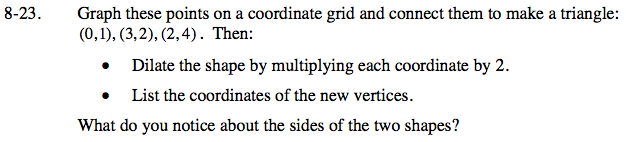Home > ACC7 > Chapter cc38 > Lesson cc38.1.2 > Problem8-23

8-23.
1. Graph these points on a coordinate grid and connect them to make a triangle: (0, 1), (3, 2), (2, 4). Then:

• • Dilate the shape by multiplying each coordinate by 2.

• • List the coordinates of the new vertices.

2. What do you notice about the sides of the two shapes? 8-23 HW eTool (Desmos). Homework Help ✎Look at the graph to find the coordinates of the new vertices. What do you notice about the sides of the triangles?

All pairs of corresponding sides are parallel.

Use the eTool below to complete the problem.
Click the link at right for the full version of the eTool: CC3 8-23 HW eTool